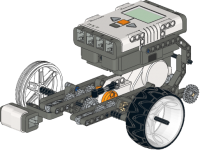## Overview

ANSYS Mechanical is a finite element analysis tool for structural analysis, including linear, nonlinear and dynamic studies. Thiscomputer simulation product provides finite elements to model behavior, and supports material models and equation solvers for a wide range of mechanical design problems. ANSYS Mechanical also includes thermal analysis and coupled-physics capabilities involving acoustics, piezoelectric, thermal–structural and thermo-electric analysis.

## Course Details

1. Introduction to the CAD, CAM, CAE Software.
2. Introduction to the Concept of Fluids.
3. Fundamentals of Aero Dynamics.
4. Aerodynamics of Formula 1 and Flights.
5. Effects of Air on the Structures.
6. Introduction to Computational Fluid Dynamics.
7. Calculation of the Effect of Air with the ANSYS Software.
8. Introduction to Using ANSYS Fluent in ANSYS Workbench: Fluid Flow and Heat Transfer in a Mixing Elbow

&nbsb;

• Introduction
• Prerequisites
• Problem Description
• Setup and Solution
• Preparation
• Creating a Fluent Fluid Flow Analysis System in ANSYS Workbench
• Creating the Geometry in ANSYS Design Modeler
• Meshing the Geometry in the ANSYS Meshing Application
• Setting Up the CFD Simulation in ANSYS Fluent
• Displaying Results in ANSYS Fluent and CFD-Post
• Duplicating the Fluent-Based Fluid Flow Analysis System
• Changing the Geometry in ANSYS Design Modeler
• Updating the Mesh in the ANSYS Meshing Application
• Calculating a New Solution in ANSYS Fluent
• Comparing the results of both system in CFD-Post
• Summary

### 1. Parametric Analysis in ANSYS Workbench Using ANSYS Fluent

• Introduction
• Prerequisites
• Problem Description
• Setup and Solution
• Preparation
• Adding Constraints to ANSYS Design Modeler Parameters in ANSYS Workbench
• Setting Up the CFD Simulation in ANSYS Fluent
• Defining Input Parameters in ANSYS Fluent and Running the Simulation
• Post processing and Setting the Output Parameters in ANSYS CFD-Post
• Creating Additional Design Points in ANSYS Workbench
• Post processing the New Design Points in CFD-Post
• Summary

### 1. Introduction to Using ANSYS Fluent: Fluid Flow and Heat Transfer in a Mixing Elbow

• Introduction
• Prerequisites
• Problem Description
• Setup and Solution
• Preparation
• Launching ANSYS Fluent
• Reading the Mesh
• General Settings
• Models
• Materials
• Cell Zone Conditions
• Boundary Conditions
• Solution
• Displaying the Preliminary Solution
• Using the Coupled Solver
• Adapting the Mesh

### Finally Projects to be covered

• Making a simple vertical wind mill and performing its analysis for any velocity of wind.
• Liquid flow analysis in in simple pipe with a nozzle
• Spring mass system analysis
• Sandwich laminated beam model analysis for vibration
• Truss analysis of simple suspension system.

## Our Technologies